Next: A Hierarchy of Geometries Up: Recognition with Invariants Previous: Generalities

## Five Coplanar Points

Now consider the simple case of 5 coplanar points in general position. This example will show that although the theory provides a nice framework, it does not give all the answers.

The isotropy subgroup is the identity: even four of the points are sufficient to define a unique projective basis. There are therefore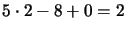independent projective invariants. In section 3.1.2, we showed that two suitable invariants can be obtained by taking cross ratios of pencils of lines through any two of the points.

Ideally, we would like to be able to recognise one among a set of such configurations by computing the two cross ratios from image data and searching a database for the closest known configuration with those invariant values. This raises two questions:
1) Which of the 5!=120 possible orderings of the image points was used for the invariant stored in the database?
2) How should proximity of cross ratios be measured?

The first point is a combinatorial/correspondence problem. One way to attack this is to create combinations of invariants that are unchanged under permutations of the points. For example, the 6 possible values of a cross ratio can be combined into a symmetric, order independent form such as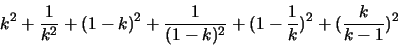Exercise 3.10   : The simplest symmetrical polynomials would be the sum or product of all the values. Why are these not useful?

Even given this, we still have to choose two of the five points as base points for the symmetrized cross ratios. Again we can form some symmetric function of the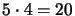possiblities. We could take the maximum or minimum, or some symmetric polynomial such as: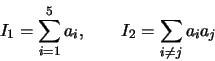The problem with this is that each time we symmetrize the invariants lose discriminating power.

To compare invariants, something like the traditional Mahalanobis distance can be used. However, most projective invariants are highly nonlinear, so the distance has to be evaluated separately at each configuration: using a single overall distance threshold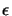usually gives very bad results. In fact, close to degenerate configurations -- here, when three of the points are almost aligned -- even well-designed Mahalanobis metrics may be insufficient. Such configurations have to be processed case-by-case (usually, they are discarded).

Hence, even with careful design, the results of this indexation approach are not very satisfactory. An exhaustive test on randomly generated point configurations was performed in , with the conclusion that no usable trade off between false positives and false negatives existed.

However, the results can be improved by several order of magnitude by the following process:

1.
Given that convexity and order is preserved under perspective image projections (although not under general projective mappings!), classify the 5 point configurations into one of the three classes described in fig. 3.6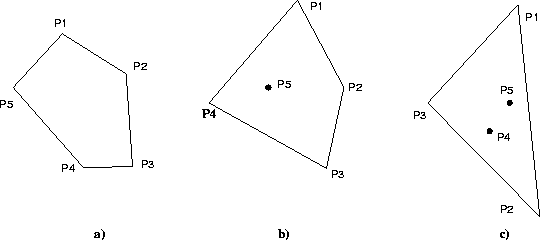2.
For each class, compute the possible cross ratios. For instance, for class (a) there are five possibilities for the five vertices of the polygon, with the points considered in clockwise order.
3.
For each set of (redundant) invariants, compute the Mahalanobis distance, index, and perform final classification by projective alignment with each of the retrieved candidate configurations.

Ordering the points allows the use of raw (non-symmetrized) cross ratios, which significantly improves the discriminating power. It also makes the invariant computation specific to each class of object considered. With random noise of 1.5 pixel in a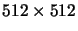image, this process prunes on average about 799 of every 800 configurations. These results have also been validated by independent experiments .Next: A Hierarchy of Geometries Up: Recognition with Invariants Previous: Generalities
Bill Triggs
1998-11-13